# Class 12 Work sheet derivative to find tangents and normals

5698

Mathematics Integration Level: Misc Level

Find the slopes of the tangents at the three points where the curve cuts the x-axis.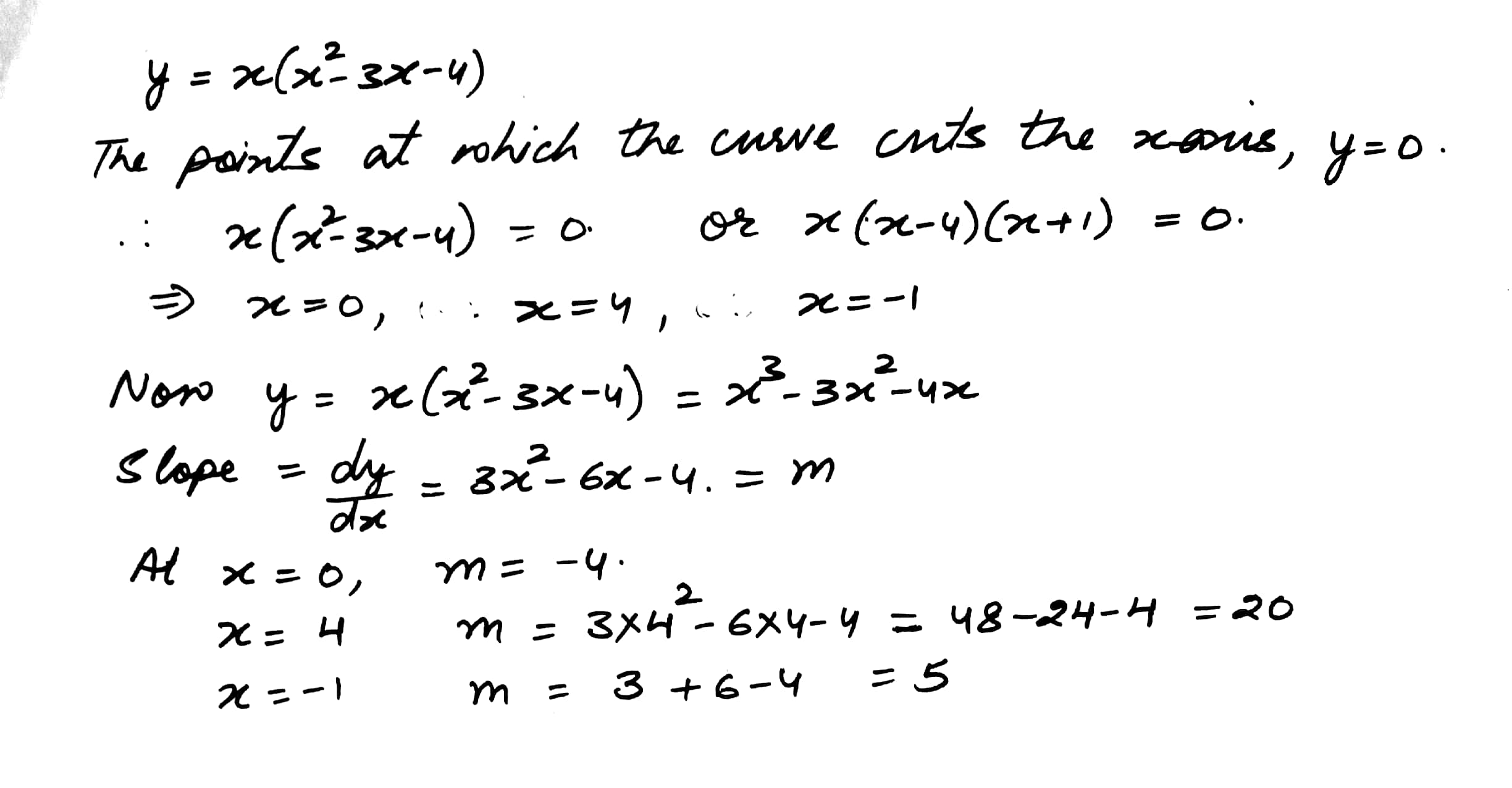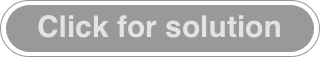5699

Mathematics Integration Level: Misc Level

Show that the tangents to the curve at the points where x=2 and x=-2 are parallel.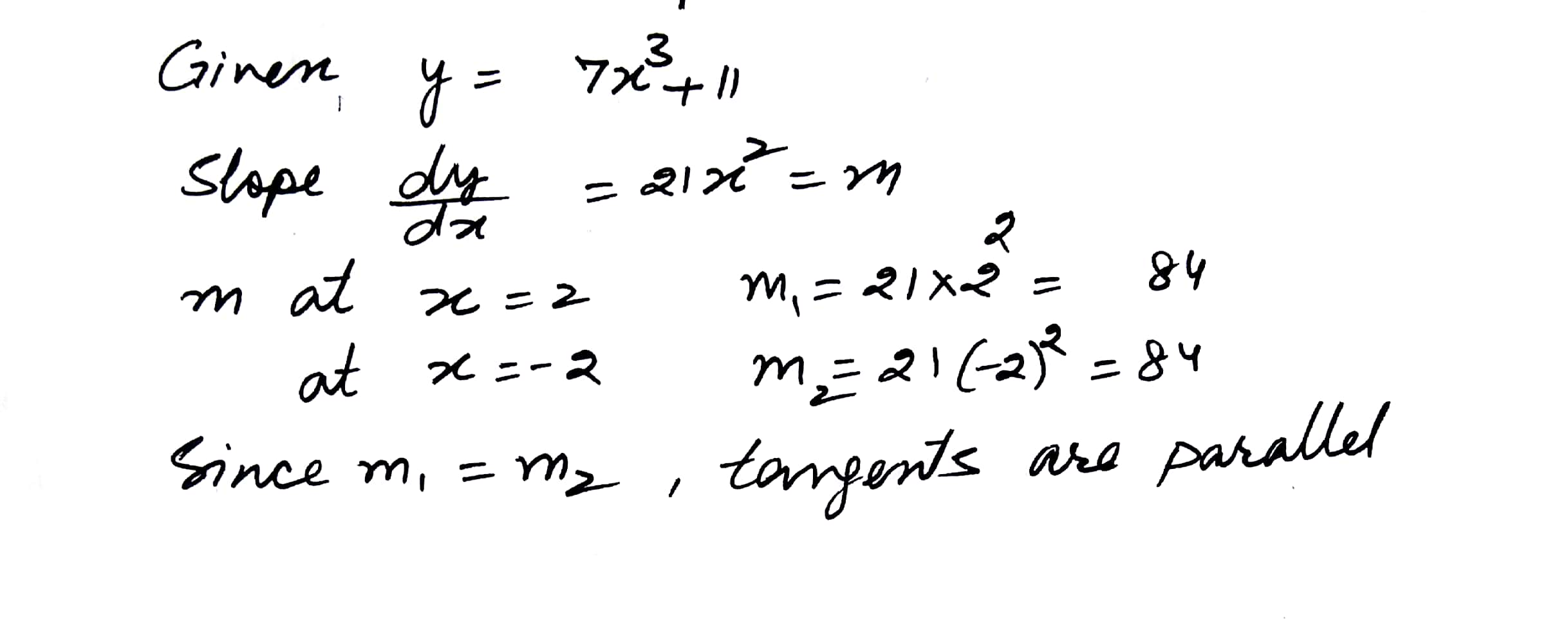5700

Mathematics Integration Level: Misc Level

If the tangent to the curve y=x3++ax+b at (1,-6) is parallel to the line 2x-2y+7=0, find a anb b.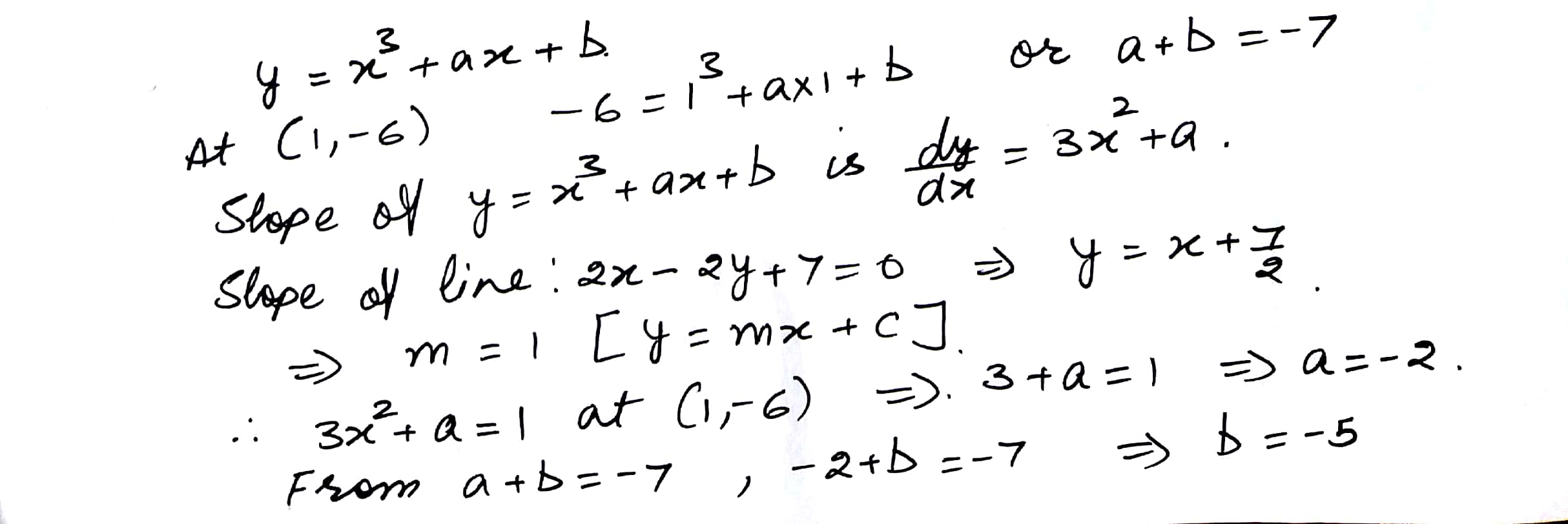5701

Mathematics Integration Level: Misc Level

Determine the point of the curve y=3x2-5 at which the tangent is perpendicular to the line whose slope is -1/3.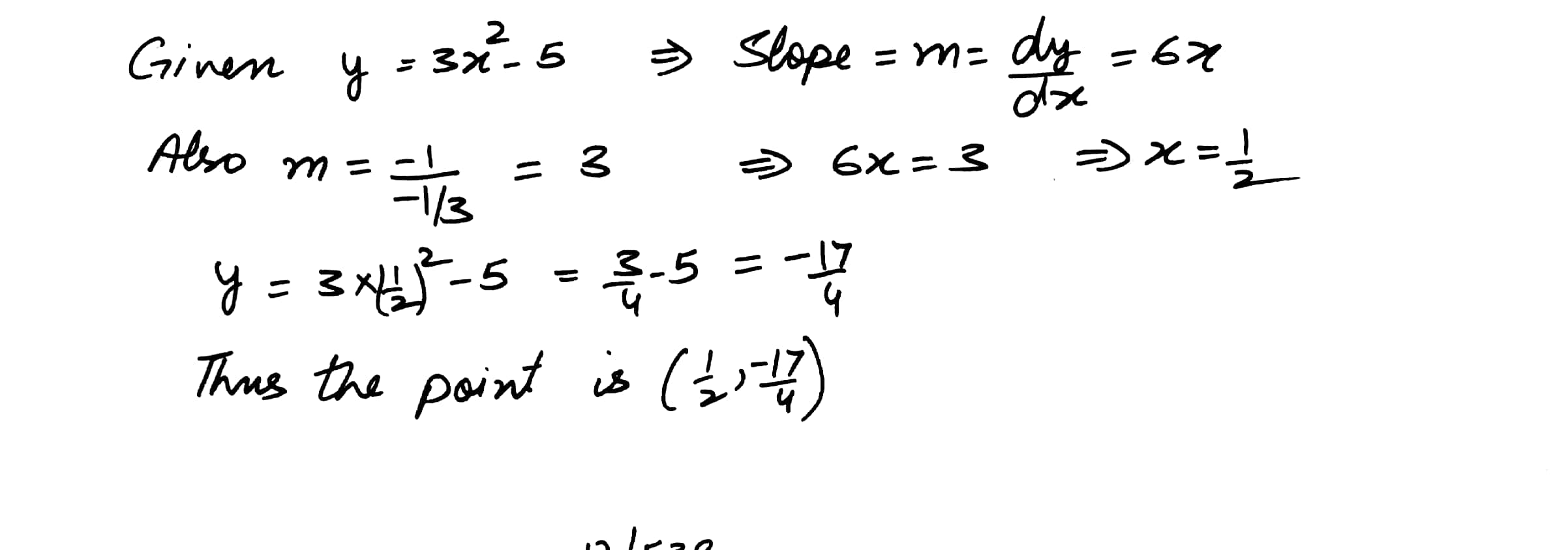5702

Mathematics Integration Level: Misc Level

Find the points on the curve at which the tangents are.

(a)  parallel to x-axis

(b)  parallel to y-axis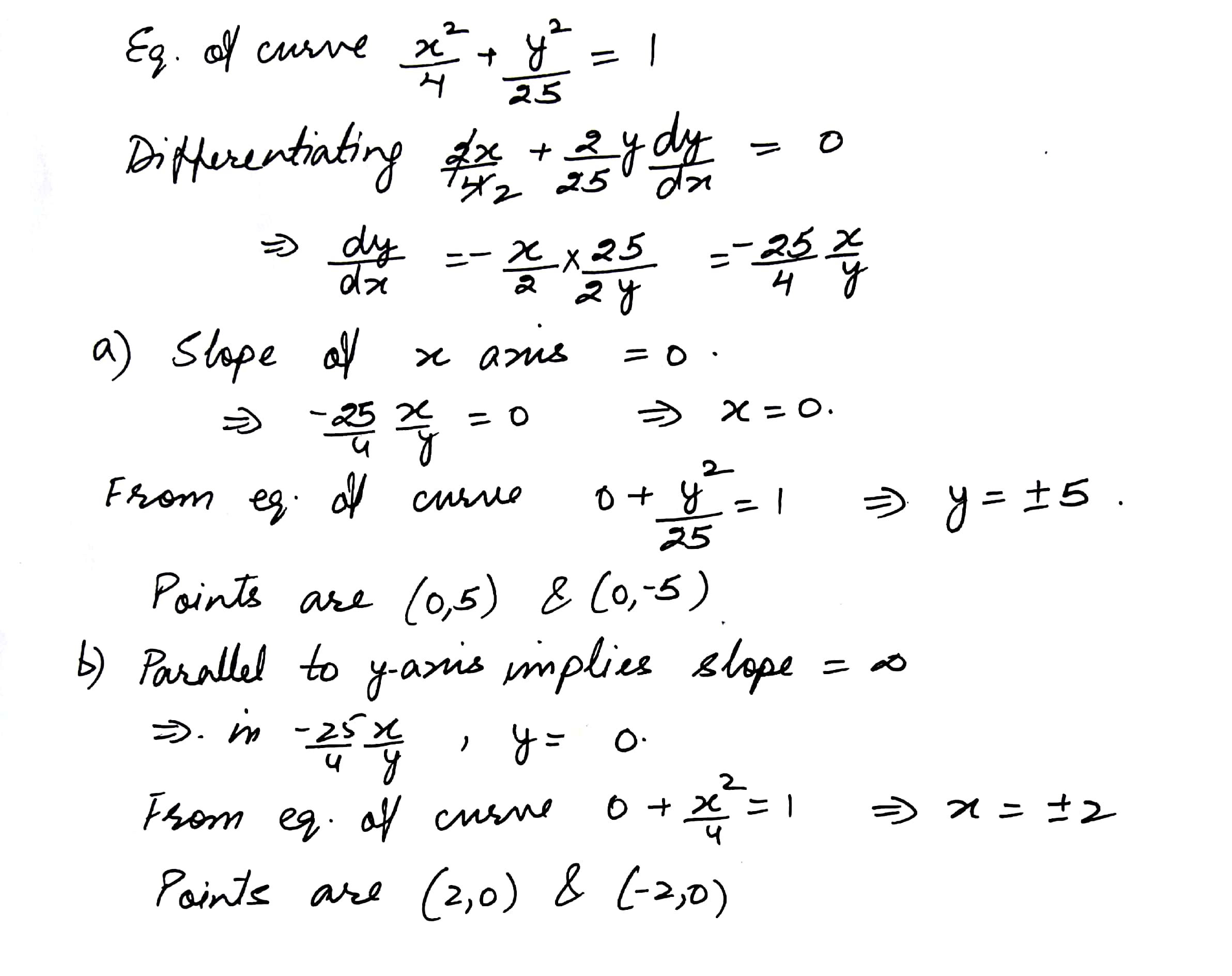5703

Mathematics Integration Level: Misc Level

Find the equations of the tangent and the normal to the curve y = x4 - 6x3+13x2 - 10x + 5 at (1,3).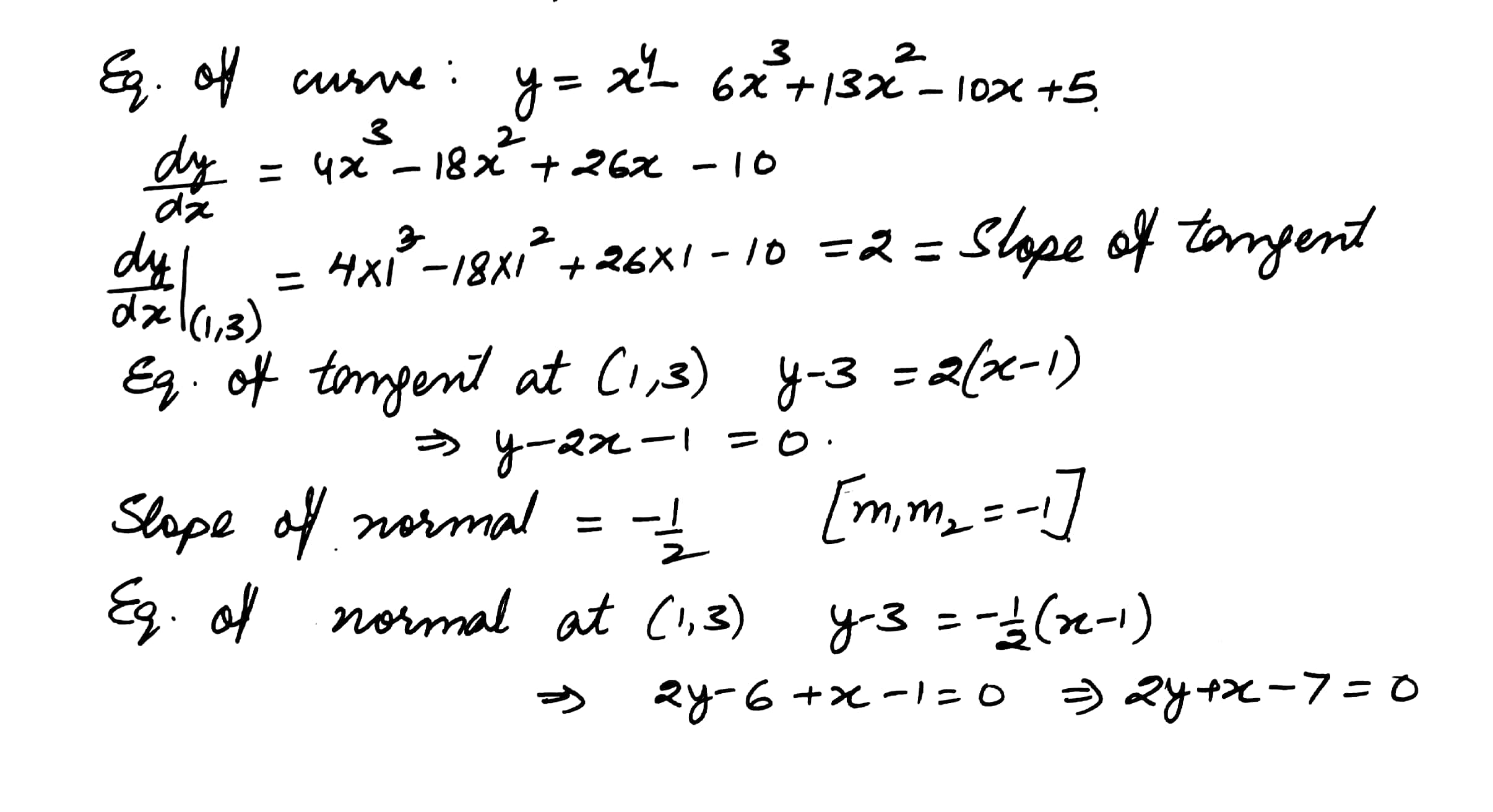5704

Mathematics Integration Level: Misc Level

Find the equations of the tangent and the normal to the curve   at (1,1).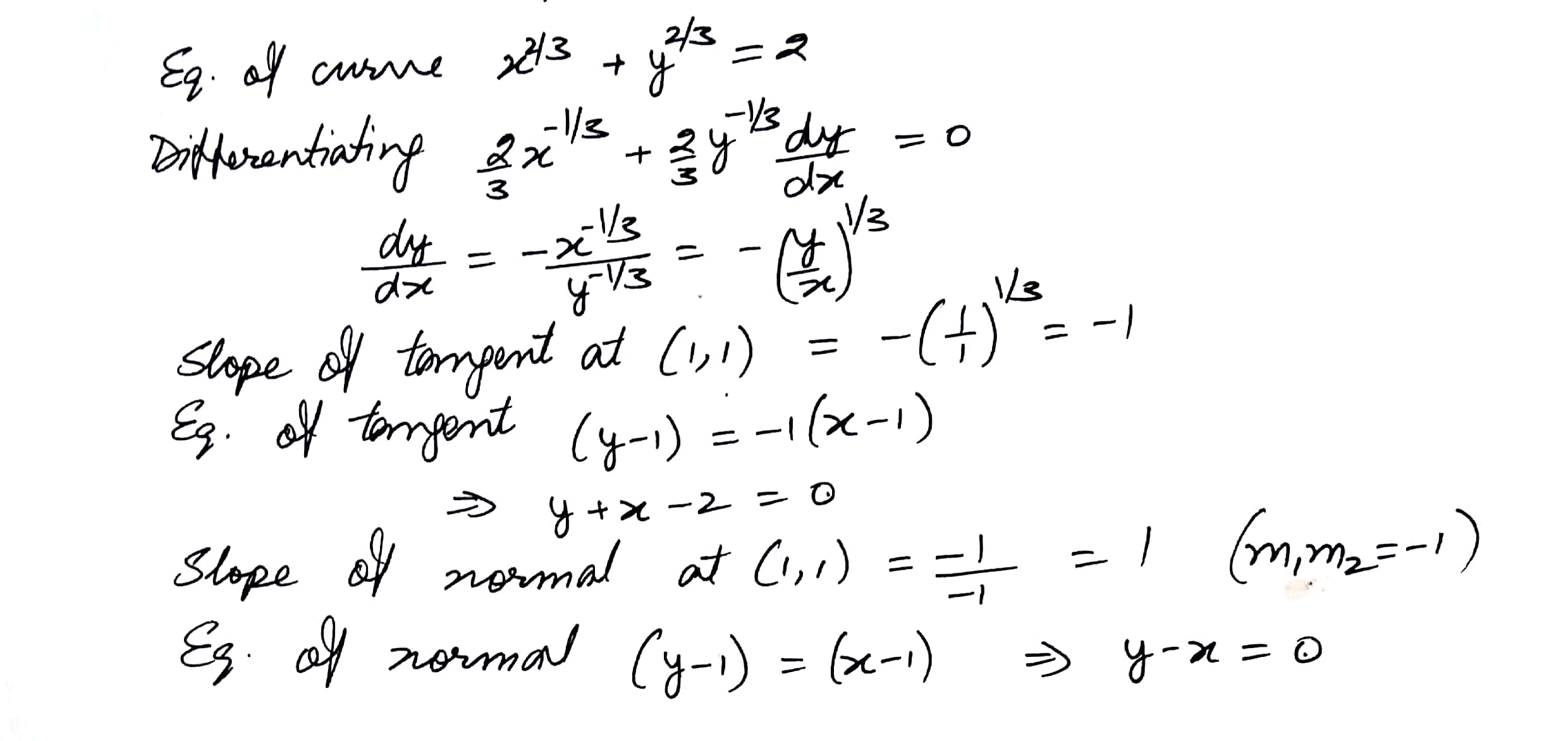5705

Mathematics Integration Level: Misc Level

Find the equations of the tangent to the curve x = Sin 3t, y = Cos 2t at .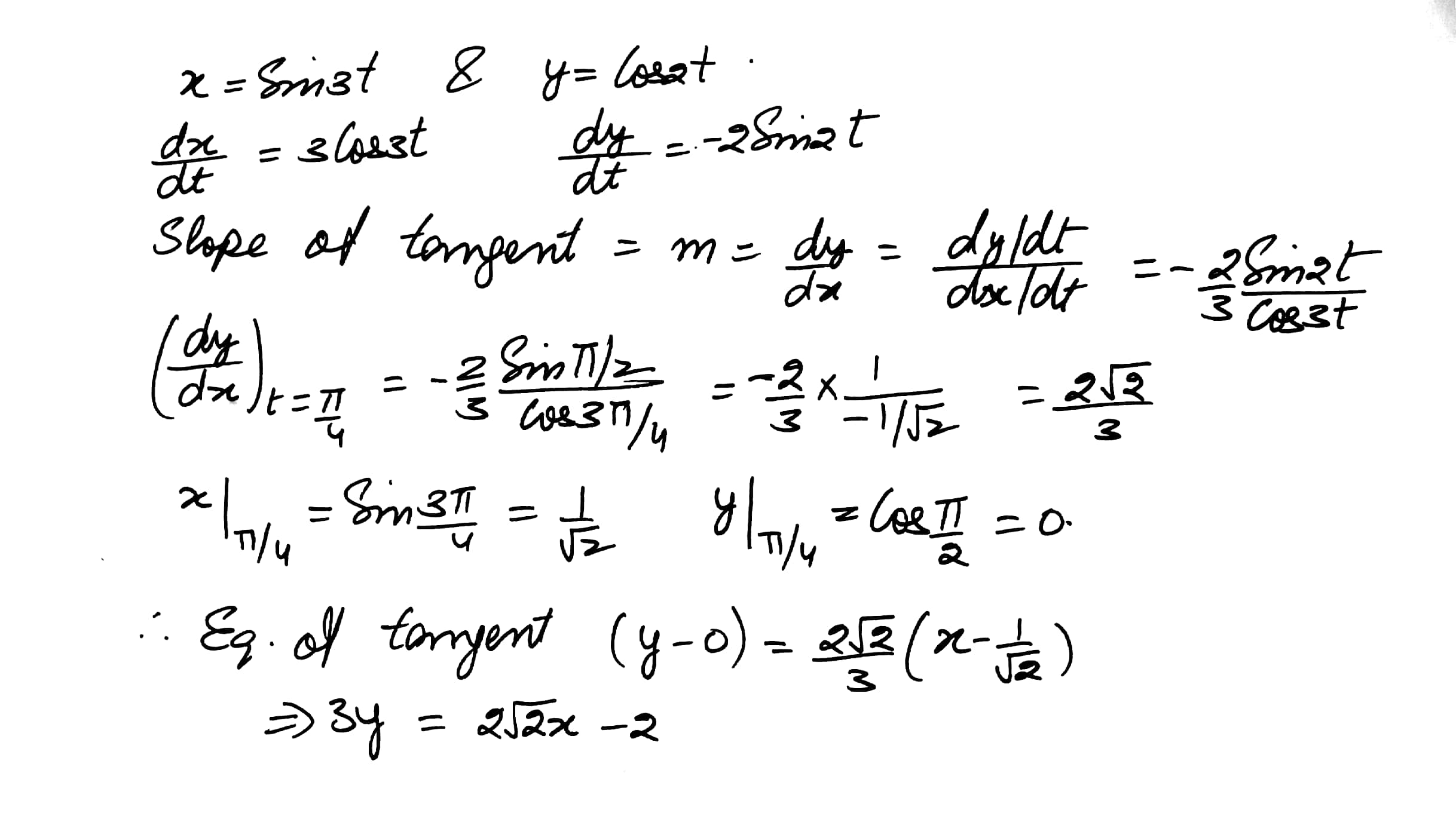5706

Mathematics Integration Level: Misc Level

Find the equations of all lines having slope 2 and that are tangents to the curve .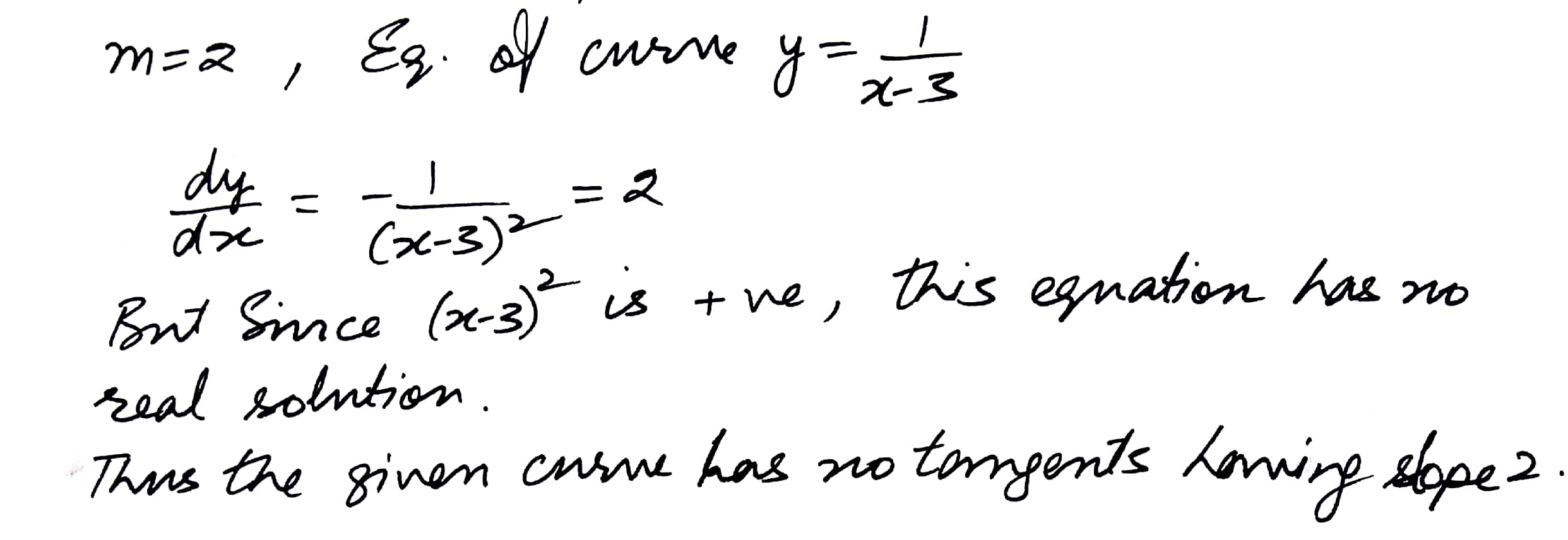5707

Mathematics Integration Level: Misc Level

Find the equations of the tangents to the curve y = x3 + 2x - 4 which are perpendicular to theline x + 14y + 3 = 0.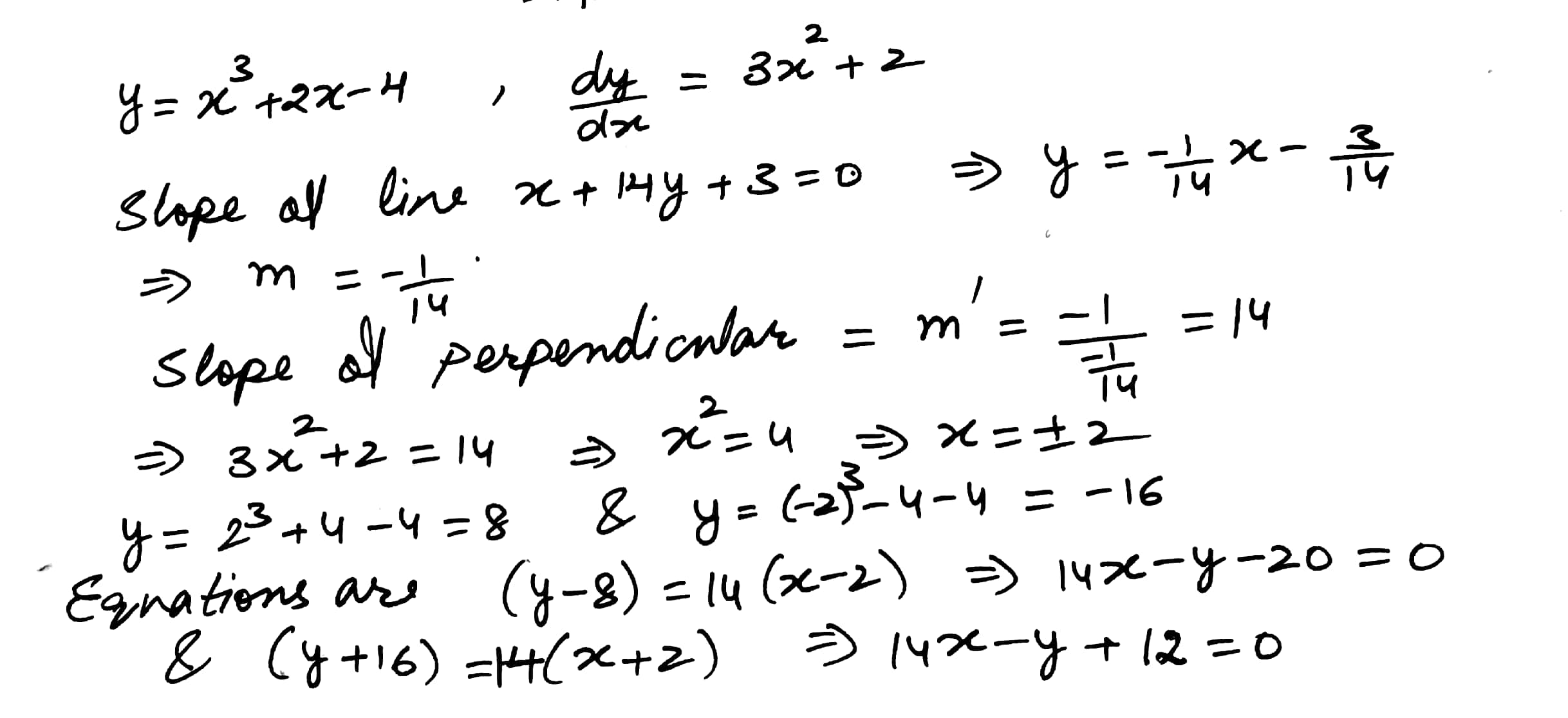Displaying 1-10 of 12 results.